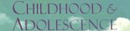Encyclopedia Article

# Average - Research Article from Encyclopedia of Childhood and Adolescence

This encyclopedia article consists of approximately 1 page of information about Average.
 This section contains 266 words (approx. 1 page at 300 words per page)

One measure of central tendency in statistics.

The term average is popularly used to refer to a value that is typical of a group. For example, a person may be described as being of average height or average intelligence. Educators and school administrators may describe the average for the population of students in their school. Average always describes a relative value; for example, the average score on a standardized test for students in a particular class may or may not equal the average score for all students in the school. Yet another value will be the average for all test-takers nationwide. Thus, the average is often used as a way to compare attributes of two different groups.

Average is one of the measures of central tendency used in statistics. There are three precise measures of central tendency calculated by statisticians when studying sets of data. The mean is calculated by adding together all the numbers in the set being studied, and dividing the total by the number of data points. For example, if the statistician is calculating the mean test score for a group of 27 test-takers, he would add together the scores of all 27 people, and divide the total by 27. Grade-point average, calculated by adding all the numerical values for a student's grades together and dividing by the number of grades received, is an example of a mean. The median, or midpoint, for the set of test scores would be the score precisely at the midpoint when the scores are ranked in numerical order. The mode is the score that was achieved most often.

 This section contains 266 words (approx. 1 page at 300 words per page)
More summaries and resources for teaching or studying Average.